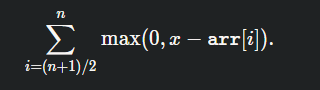# Solving Binary Search on Answer Problems

I’m working on Silver>Misc.Topics>Binary Search on Answer: Binary Search on the Answer · USACO Guide

On there, for the Maximum Median problem, I’m confused about how they derived the following summation:To rephrase, I know that it works, but how did you think of that equation?

Here is the link to the Maximum Median Problem: Binary Search on the Answer · USACO Guide.

The solution on USACO guide, first sorts the array. Then basically tries different maximum median values. They deem if a median value is plausible by using the above equation. They then try a new maximum medium value, by binary searching it (which makes sense)

So just to be clear, you understand how and why, but not how the motivation for deriving that equation, correct?

Sorry, I don’t understand how it works either. I plugged in a few numbers so I know it works, but I don’t know how it works. I also don’t know the motivation in deriving the equation.

Thanks!

Median

The value at (n + 1) / 2 of a sorted array. What does that mean about the median relative to the other elements in the sorted array?

Hopefully, more thought will help you understand this better. Repost if you are still stuck, and mention what part doesn’t make sense to you. The motivation for deriving this equation comes from what a median is.

Thanks for the hint. Okay so logically thinking about it, I want to increase the median value of the array. Therefore, I only have to change the values from the median index up, the initial values don’t matter. This explains why the summation starts at (n+1)/2 the median.

Ohh so regarding the x-arr[i] stuff, if I want to increase the median to x, I’ll have to increase the value at the medium index up to all just x. Therefore, everything is x- arr[i].

Then the max(0, …) part is there to make sure any values greater than x, arent needly increased. And so that the summation doesn’t have any negative values.

Okayyy so from that hint, I can see why and how the equation works. But if I was doing this in contest, I wouldn’t be able to derive that, it seems advanced. Can you please advise me on how I can initially think of this solution?

Thanks.

In an actual contest, I would focus on thinking about the problem more. Understanding what the problem is referring to and what is given in the problem is really important for solving these problems. You should also consider the time complexity given by N. I think the main problem was that you didn’t spend enough time thinking, so you didn’t really pay attention to the details of the problem. In a USACO contest, you are allotted an hour and 20 minutes per problem (on average for non-open contests); this allows you to think more about this problem. However, in a CF contest, how fast you submit your solution is considered in the scoring. For CF problems, I would recommend you practice problems of similar difficulty and type for these. Hope that helps!

1 Like

Totally didn’t read this entire thread but I also had a hard time understanding the solution even after reading the editorial. This comment explained the question very well: Codeforces Round #577 (Div 2) Editorial - Codeforces